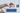# Replace all spaces in a string with %20

October 01, 2020## Problem Statement

Replace all spaces in a string with ‘%20’ (three characters). Do this in place and assume you have enough space in array

## Solution (Simple)

This is the simplest solution which many people might think.

• Iterate from left side (begining)
• After encountering a space, first shift entire array by 2 places.
• Put `%20` at consecutive location
• Repeat this process

Obviously, if you see you need to shift the remaining array as many times as number of spaces. And, is not an efficient algorithm.

I will not put this silly code.

### Complexity

The complexity would be close to `O(n^2)`

## A Better Solution

If you start from left side, you saw we need to shift the array. What if we start from the right side.

• First calculate number of spaces in array, this will help in calculating the resultant last index
• Start iterating from right most side.
• Maintain two index counters. One for original array characters, other for resultant index positions.
• Start copying character one by one.
• If found space, just copy consecutive `%20`

At the end, you will achieve the purpose. Lets see the code

``````private static int countSpaces(char[] input) {
int c = 0;
for (int i=0; i<input.length; i++) {
if (input[i] == ' ') {
c ++;
}
}
return c;
}

public static int replace(char[] input, int len) {
int spaces = countSpaces(input);
if (spaces == 0) return len;

int lastIndex = (len-1) + (2 * spaces);
int resultIndex = lastIndex;

for (int i=len-1; i>=0; i--) {
if (input[i] == ' ') {
//copy %20
input[lastIndex] = '0';
input[lastIndex-1] = '2';
input[lastIndex-2] = '%';
lastIndex -= 3;
}
else {
//copy actual characters
input[lastIndex] = input[i];
lastIndex --;
}
}

return resultIndex;
}``````

### Complexity

The complexity is `O(n)`